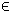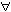## 5MIQP Problem

The general formulation in TOMLAB for a mixed-integer quadratic programming problem is:

 min x
f(x) =
1
2
xT F x + cT x

s/t
 xL ≤ x ≤ xU, bL ≤ A x ≤ bU,  xjNjI
(6)
where c, x, xL, xURn, ARm1 × n, and bL,bURm1. The variables xI, the index subset of 1,...,n are restricted to be integers. Equality constraints are defined by setting the lower bound equal to the upper bound, i.e. for constraint i: bL(i) = bU(i).

The following file illustrates how to solve a MIQP problem in TOMLAB.

File: tomlab/quickguide/miqpQG.m

Open the file for viewing, and execute miqpQG in Matlab.
```% miqpQG is a small example problem for defining and solving
% mixed-integer quadratic programming problems using the TOMLAB format.

c    = [-6 0]';
Name = 'XP Ref Manual MIQP';
F    = [4 -2;-2 4];
A    = [1 1];
b_L  = -Inf;
b_U  = 1.9;
x_L  = [0 0]';
x_U  = [Inf Inf]';

% Defining first variable as an integer
IntVars   = 1;

% Assign routine for defining a MIQP problem.
Prob = miqpAssign(F, c, A, b_L, b_U, x_L, x_U, [], ...
IntVars, [], [], [], Name, [], []);

% Calling driver routine tomRun to run the solver.
% The 1 sets the print level after optimization.

Result = tomRun('cplex', Prob, 1);
%Result = tomRun('oqnlp', Prob, 1);
%Result = tomRun('miqpBB', Prob, 1);
%Result = tomRun('xpress-mp', Prob, 1);
%Result = tomRun('minlpBB', Prob, 1);```